## Classification

# of variables # of modes # of jumps
n 3 2
Type Continuous dynamics Guards & Invariants Resets
hybrid non-polynomial linear polynomial identity

Note: The class of the benchmarks is determined by the input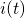.

 Flow* n=2 line_circuit_2.model Flow* n=2 line_circuit_4.model Flow* n=2 line_circuit_6.model Flow* n=2 line_circuit_8.model Flow* n=2 line_circuit_10.model Flow* n=2 line_circuit_12.model

## Model description

We study a non-linear resistor circuit which is shown in the figure below.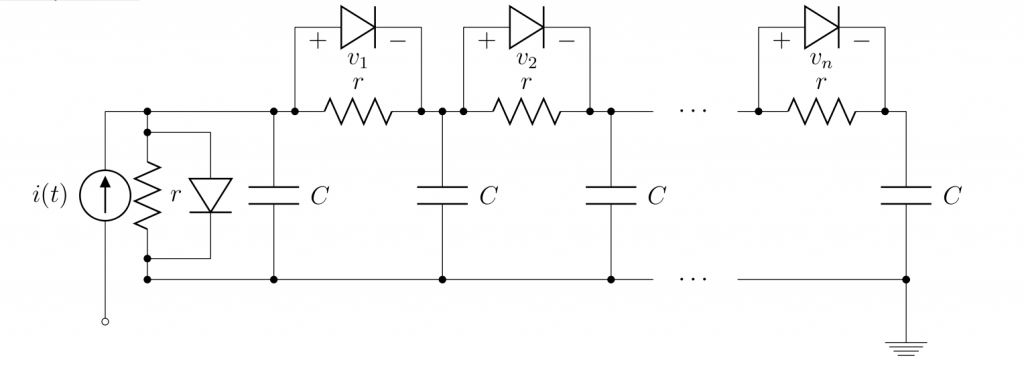The circuit is composed of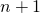non-linear resistors and the same number of capacitors. Each non-linear resistor consists of a diode and a unit resistor (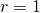). For simplicity, we assume that all capacitors have unit capacitance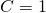. For each diode, the I-V characteristic is given by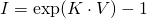. The value of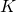is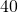in the original model, but we set it as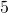to relieve the stiffness of the behavior. The current sourcein the figure is the input, and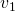is the single output of the circuit. Therefore, the whole circuit system can be described by the following ODE.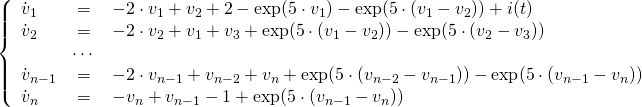Scalable continuous and hybrid benchmarks can be built based on the circuit according to the size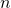and various types of the input. Here, we consider a discontinuous inputwhich is defined by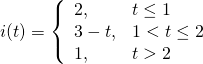## Reachability settings

The initial set under consideration is defined by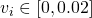for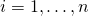.

## Results

The following figure shows an overapproximation of the reachable set for the case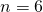over the time horizon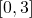computed by Flow*: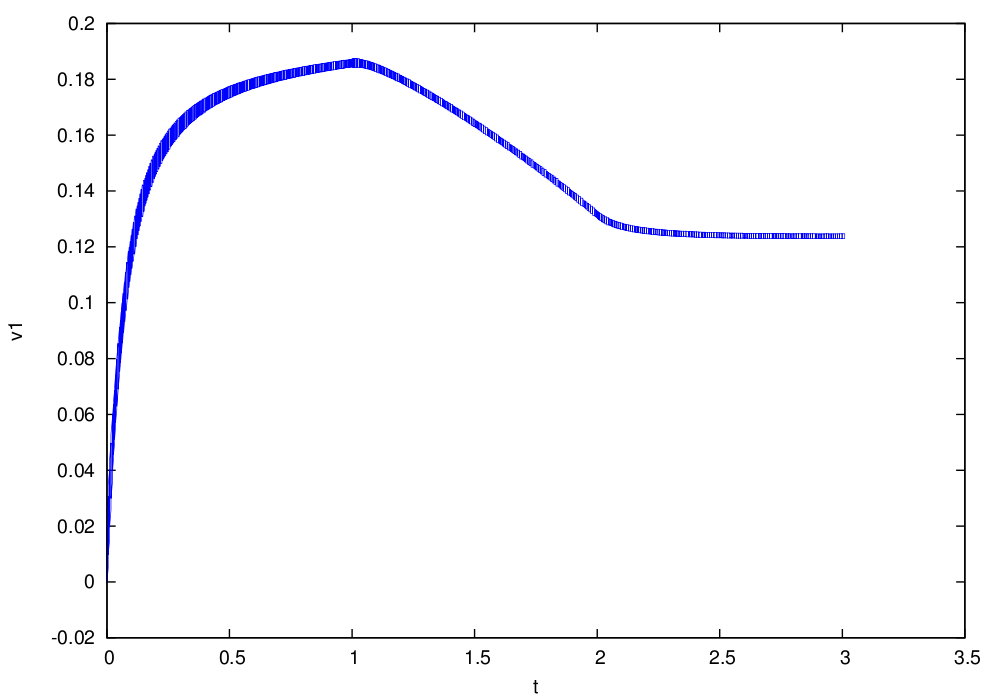## References

 M. Rewienski, J. White. A trajectory piecewise-linear approach to model order reduction and fast simulation of nonlinear circuits and micromachined devices. In IEEE Transactions on Computer-Aided Design of Integrated Circuits and Systems, Volume 22(2), pages 155–170, IEEE, 2003.